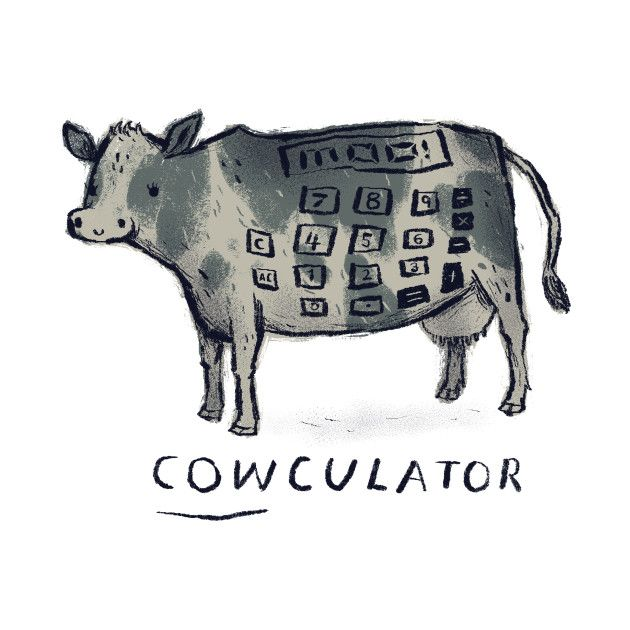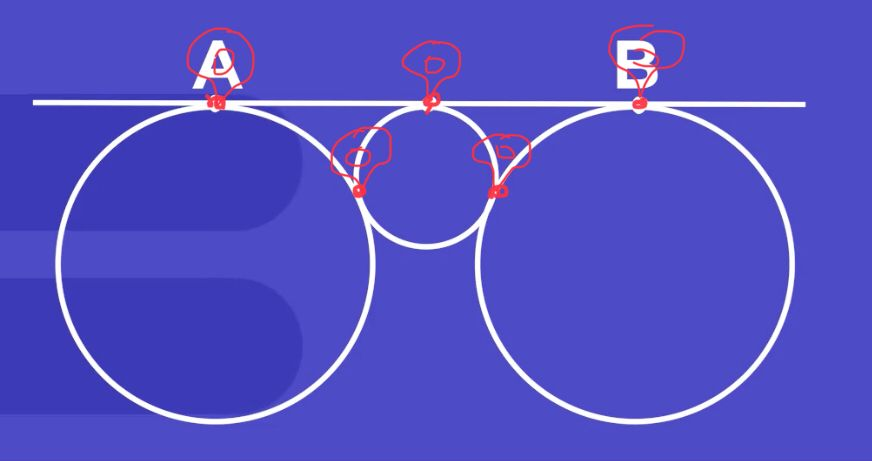# Maths Jokes 😄

• How do we know that $$\frac{p}{c}$$, $$\frac{o}{c}$$, $$\frac{s}{c}$$, $$\frac{h}{c}$$, $$\frac{e}{c}$$, $$\frac{n}{c}$$, $$\frac{l}{c}$$, $$\frac{o}{c}$$, $$\frac{h}{c}$$ are all in Antarctica?
Because they are all over-c’s!PS I chose Antarctica because I’m pretty sure no one here on the Forum lives there. If I chose something like, say, Canada, then the joke won’t be inclusive.

• Why did Po-Shen the mathematicianand Loh the bakerhave an argument?
Because the mathematicianthinks $$\pi r^{2}$$ and the bakerthinks pies are round.

🥧🧁

• @RZ923 This article claims that starting from a single female bunny, after seven years, you would get 94,862,569,280 rabbits!

http://www.bio.miami.edu/hare/scary.html• How do we know that $$\frac{p}{c}$$, $$\frac{o}{c}$$, $$\frac{s}{c}$$, $$\frac{h}{c}$$, $$\frac{e}{c}$$, $$\frac{n}{c}$$, $$\frac{l}{c}$$, $$\frac{o}{c}$$, $$\frac{h}{c}$$ are all in Antarctica?
Because they are all over-c’s!PS I chose Antarctica because I’m pretty sure no one here on the Forum lives there. If I chose something like, say, Canada, then the joke won’t be inclusive.

You'd be surprised to know how wrong you areOk it's more temporary, although a few months at a time seems pretty long for me

• Student: “Teacher, will I have any problems on the maths exam?”
Teacher: ”I think the exam is all problems...”
🧮️♾•• @RZ923 Sooooooo funny!!You guys are so clever!• Why is algebra so easy for the Romans?
X is always equal to 10.

$$x=10$$

• Why did the chicken cross the Möbius strip?To get to the same side.

• What do you call a number that can't stay in one place?

A Roamin' Numeral• This post is deleted!

• What do you call a Prof Lohafter he went to the beachand came back to design a course of circle️ geometry?
A tan-gent.• This thread has succeeded the popularity of the green shirt petitionWow• Doing AoPS, Daily Challenge, and Areteem:I think it should say person not just man but unfortunately that's the way it is

• @The-Blade-Dancer lol i can relate

• Are fictional charactersgood at maths?
Not unless you Count Dracula.
🧛 🧮

• I saw my math teacher with a piece of graph paper yesterday. I think he must be plotting something.• To post more maths jokes, go to Math Jokes Part II!

PS @debbie plz lock this topic.